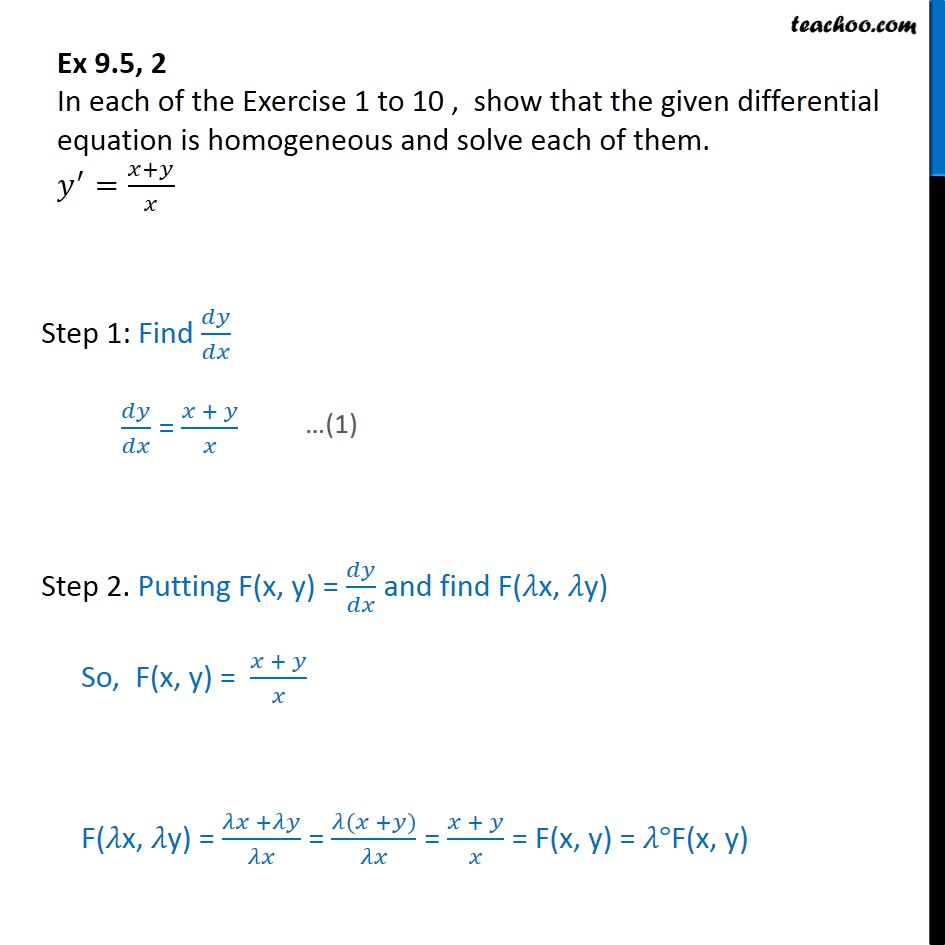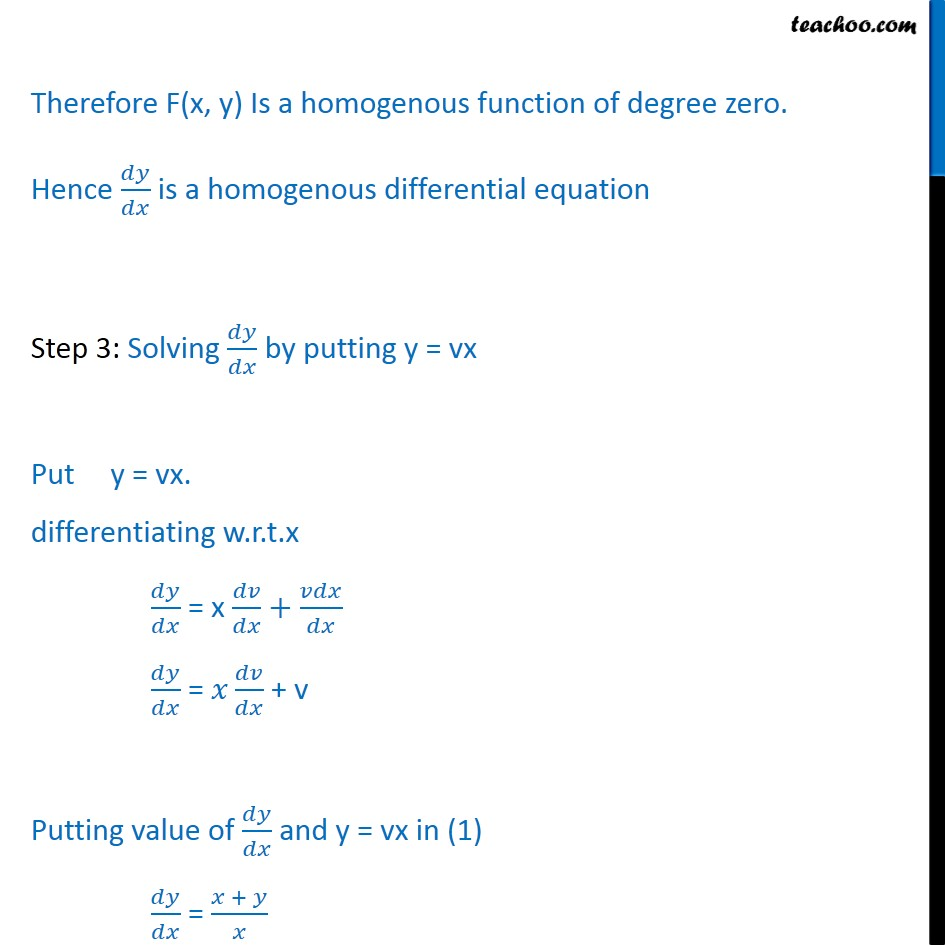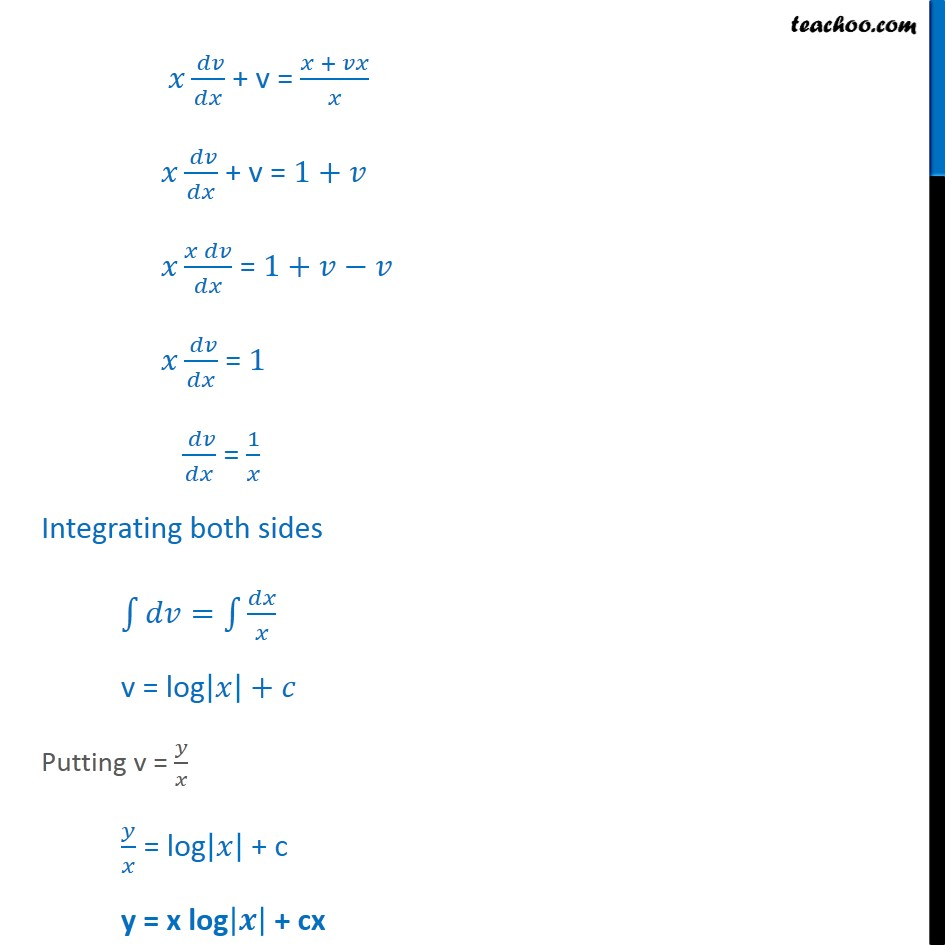Ex 9.4

Chapter 9 Class 12 Differential Equations
Serial order wiseLearn in your speed, with individual attention - Teachoo Maths 1-on-1 Class

### Transcript

Ex 9.4, 2 In each of the Exercise 1 to 10 , show that the given differential equation is homogeneous and solve each of them. 𝑦^′=(𝑥+𝑦)/𝑥 Step 1: Find 𝑑𝑦/𝑑𝑥 𝑑𝑦/𝑑𝑥 = (𝑥 + 𝑦)/𝑥 Step 2. Putting F(x, y) = 𝑑𝑦/𝑑𝑥 and find F(𝜆x, 𝜆y) So, F(x, y) = (𝑥 + 𝑦)/𝑥 F(𝜆x, 𝜆y) = (𝜆𝑥 +𝜆𝑦)/𝜆𝑥 = (𝜆(𝑥 +𝑦))/𝜆𝑥 = (𝑥 + 𝑦)/𝑥 = F(x, y) = 𝜆°F(x, y) Therefore F(x, y) Is a homogenous function of degree zero. Hence 𝑑𝑦/𝑑𝑥 is a homogenous differential equation Step 3: Solving 𝑑𝑦/𝑑𝑥 by putting y = vx Put y = vx. differentiating w.r.t.x 𝑑𝑦/𝑑𝑥 = x 𝑑𝑣/𝑑𝑥+𝑣𝑑𝑥/𝑑𝑥 𝑑𝑦/𝑑𝑥 = 𝑥 𝑑𝑣/𝑑𝑥 + v Putting value of 𝑑𝑦/𝑑𝑥 and y = vx in (1) 𝑑𝑦/𝑑𝑥 = (𝑥 + 𝑦)/𝑥 𝑥 ( 𝑑𝑣)/𝑑𝑥 + v = (𝑥 + 𝑣𝑥)/𝑥 𝑥 ( 𝑑𝑣)/𝑑𝑥 + v = 1+𝑣 𝑥 (𝑥 𝑑𝑣)/𝑑𝑥 = 1+𝑣−𝑣 𝑥 ( 𝑑𝑣)/𝑑𝑥 = 1 ( 𝑑𝑣)/𝑑𝑥 = 1/𝑥 Integrating both sides ∫1▒〖𝑑𝑣=∫1▒〖𝑑𝑥/𝑥 〗 〗 v = log|𝑥|+𝑐 Putting v = 𝑦/𝑥 𝑦/𝑥 = log|𝑥| + c y = x log|𝒙| + cx